Olympiad Test: Odd One Out -1

# Olympiad Test: Odd One Out -1

Test Description

## 20 Questions MCQ Test Science Olympiad Class 7 | Olympiad Test: Odd One Out -1

Olympiad Test: Odd One Out -1 for Class 7 2023 is part of Science Olympiad Class 7 preparation. The Olympiad Test: Odd One Out -1 questions and answers have been prepared according to the Class 7 exam syllabus.The Olympiad Test: Odd One Out -1 MCQs are made for Class 7 2023 Exam. Find important definitions, questions, notes, meanings, examples, exercises, MCQs and online tests for Olympiad Test: Odd One Out -1 below.
 1 Crore+ students have signed up on EduRev. Have you?
Olympiad Test: Odd One Out -1 - Question 1

### Directions: In each problem, out of the five figures marked (1), (2), (3), (4) and (5), four are similar in a certain manner. However, one figure is not like the other four. Q. Choose the figure which is different from the rest.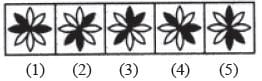Detailed Solution for Olympiad Test: Odd One Out -1 - Question 1

All other figures can be rotated into each other.

Olympiad Test: Odd One Out -1 - Question 2

### Directions: In each problem, out of the five figures marked (1), (2), (3), (4) and (5), four are similar in a certain manner. However, one figure is not like the other four. Q. Choose the figure which is different from the rest.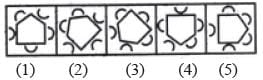Detailed Solution for Olympiad Test: Odd One Out -1 - Question 2

In each one of the figures except figure (2), three cups open towards the pentagon and two cups open outwards.

Olympiad Test: Odd One Out -1 - Question 3

### Directions: In each problem, out of the five figures marked (1), (2), (3), (4) and (5), four are similar in a certain manner. However, one figure is not like the other four. Q. Choose the figure which is different from the rest.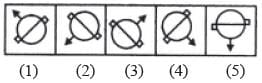Detailed Solution for Olympiad Test: Odd One Out -1 - Question 3

All other figures can be rotated into each other.

Olympiad Test: Odd One Out -1 - Question 4

Directions: In each problem, out of the five figures marked (1), (2), (3), (4) and (5), four are similar in a certain manner. However, one figure is not like the other four.

Q. Choose the figure which is different from the rest.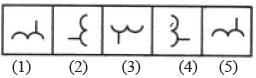Detailed Solution for Olympiad Test: Odd One Out -1 - Question 4

All other figures can be rotated into each other.

Olympiad Test: Odd One Out -1 - Question 5

Directions: In each problem, out of the five figures marked (1), (2), (3), (4) and (5), four are similar in a certain manner. However, one figure is not like the other four.

Q. Choose the figure which is different from the rest.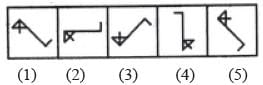Olympiad Test: Odd One Out -1 - Question 6

Directions: In each problem, out of the five figures marked (1), (2), (3), (4) and (5), four are similar in a certain manner. However, one figure is not like the other four.

Q. Choose the figure which is different from the rest.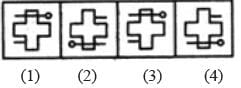Detailed Solution for Olympiad Test: Odd One Out -1 - Question 6

Only in figure (5), the small line segment lies on the same side (right side) of the main figure as the head of the pin (or all other figures can be rotated into each other).

Olympiad Test: Odd One Out -1 - Question 7

Directions: In each problem, out of the five figures marked (1), (2), (3), (4) and (5), four are similar in a certain manner. However, one figure is not like the other four.

Q. Choose the figure which is different from the rest.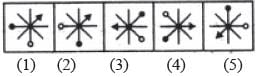Detailed Solution for Olympiad Test: Odd One Out -1 - Question 7

All other figures can be rotated into each other.

Olympiad Test: Odd One Out -1 - Question 8

Directions: In each problem, out of the five figures marked (1), (2), (3), (4) and (5), four are similar in a certain manner. However, one figure is not like the other four.

Q. Choose the figure which is different from the rest.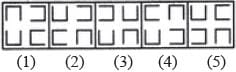Detailed Solution for Olympiad Test: Odd One Out -1 - Question 8

Only in figure (3), two out of the four elements are opening in the same direction.

Olympiad Test: Odd One Out -1 - Question 9

Directions: In each problem, out of the five figures marked (1), (2), (3), (4) and (5), four are similar in a certain manner. However, one figure is not like the other four.

Q. Choose the figure which is different from the rest.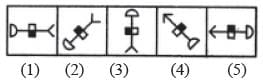Detailed Solution for Olympiad Test: Odd One Out -1 - Question 9

If all the figures are rotated so that the semicircle lies on the top, then the shaded part of the rectangle lies on the LHS.

Olympiad Test: Odd One Out -1 - Question 10

Directions: In each problem, out of the five figures marked (1), (2), (3), (4) and (5), four are similar in a certain manner. However, one figure is not like the other four.

Q. Choose the figure which is different from the rest.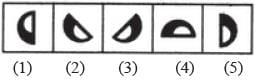Detailed Solution for Olympiad Test: Odd One Out -1 - Question 10

All other figures can be rotated into each other.

Olympiad Test: Odd One Out -1 - Question 11

Directions: In each problem, out of the five figures marked (1), (2), (3), (4) and (5), four are similar in a certain manner. However, one figure is not like the other four.

Q. Choose the figure which is different from the rest.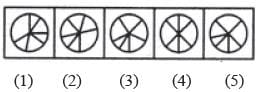Detailed Solution for Olympiad Test: Odd One Out -1 - Question 11

Only figure (4) contains three diameters of the circle.

Olympiad Test: Odd One Out -1 - Question 12

Directions: In each problem, out of the five figures marked (1), (2), (3), (4) and (5), four are similar in a certain manner. However, one figure is not like the other four.

Q. Choose the figure which is different from the rest.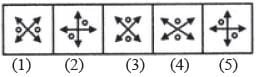Detailed Solution for Olympiad Test: Odd One Out -1 - Question 12

In all other figures, the two double sided arrows intersect each other at right angles.

Olympiad Test: Odd One Out -1 - Question 13

Directions: In each problem, out of the five figures marked (1), (2), (3), (4) and (5), four are similar in a certain manner. However, one figure is not like the other four.

Q. Choose the figure which is different from the rest.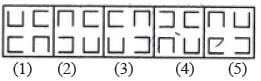Detailed Solution for Olympiad Test: Odd One Out -1 - Question 13

Only in fig. (1), two of the four elements are oriented in the same direction.

Olympiad Test: Odd One Out -1 - Question 14

Directions: In each problem, out of the five figures marked (1), (2), (3), (4) and (5), four are similar in a certain manner. However, one figure is not like the other four.

Q. Choose the figure which is different from the rest.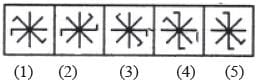Detailed Solution for Olympiad Test: Odd One Out -1 - Question 14

All other figures can be rotated into each other.

Olympiad Test: Odd One Out -1 - Question 15

Directions: In each problem, out of the five figures marked (1), (2), (3), (4) and (5), four are similar in a certain manner. However, one figure is not like the other four.

Q. Choose the figure which is different from the rest.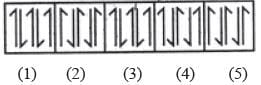Detailed Solution for Olympiad Test: Odd One Out -1 - Question 15

In all other figures, each element is obtained by rotating the adjacent element through 180o.

Olympiad Test: Odd One Out -1 - Question 16

Directions: In each problem, out of the five figures marked (1), (2), (3), (4) and (5), four are similar in a certain manner. However, one figure is not like the other four.

Q. Choose the figure which is different from the rest.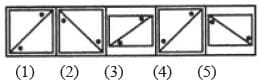Detailed Solution for Olympiad Test: Odd One Out -1 - Question 16

The two dots lie on the same side of the diagonal only in fig. (4).

Olympiad Test: Odd One Out -1 - Question 17

Directions: In each problem, out of the five figures marked (1), (2), (3), (4) and (5), four are similar in a certain manner. However, one figure is not like the other four.

Q. Choose the figure which is different from the rest.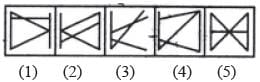Detailed Solution for Olympiad Test: Odd One Out -1 - Question 17

All other figures contain five line segments while fig. (3) contains four line segments.

Olympiad Test: Odd One Out -1 - Question 18

Directions: In each problem, out of the five figures marked (1), (2), (3), (4) and (5), four are similar in a certain manner. However, one figure is not like the other four.

Q. Choose the figure which is different from the rest.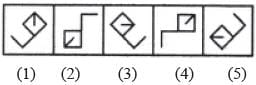Detailed Solution for Olympiad Test: Odd One Out -1 - Question 18

The line segment inside the square is attached to the corner which lies opposite to the corner to which the outer L-shaped element is attached.

Olympiad Test: Odd One Out -1 - Question 19

Directions: In each problem, out of the five figures marked (1), (2), (3), (4) and (5), four are similar in a certain manner. However, one figure is not like the other four.

Q. Choose the figure which is different from the rest.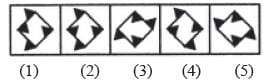Detailed Solution for Olympiad Test: Odd One Out -1 - Question 19

Only in fig. (4), each side of the square has one black triangle attached to it.

Olympiad Test: Odd One Out -1 - Question 20

Directions: In each problem, out of the five figures marked (1), (2), (3), (4) and (5), four are similar in a certain manner. However, one figure is not like the other four.

Q. Choose the figure which is different from the rest.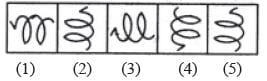Detailed Solution for Olympiad Test: Odd One Out -1 - Question 20

All other figures can be rotated into each other.